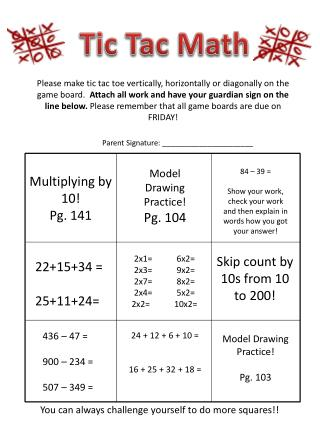DownloadDownload PresentationTic Tac Math

# Tic Tac Math

Download Presentation## Tic Tac Math

- - - - - - - - - - - - - - - - - - - - - - - - - - - E N D - - - - - - - - - - - - - - - - - - - - - - - - - - -
##### Presentation Transcript

1. Tic Tac Math Please make tic tac toe vertically, horizontally or diagonally on the game board. Attach all work and have your guardian sign on the line below. Please remember that all game boards are due on FRIDAY! Parent Signature: ______________________ Multiplying by 10! Pg. 141 Model Drawing Practice! Pg. 104 84 – 39 = Show your work, check your work and then explain in words how you got your answer! 2x1= 6x2= 2x3= 9x2= 2x7= 8x2= 2x4= 5x2= 2x2= 10x2= Skip count by 10s from 10 to 200! 22+15+34 = 25+11+24= 436 – 47 = 900 – 234 = 507 – 349 = 24 + 12 + 6 + 10 = 16 + 25 + 32 + 18 = Model Drawing Practice! Pg. 103 You can always challenge yourself to do more squares!!